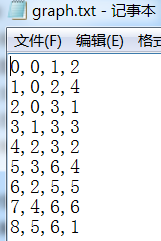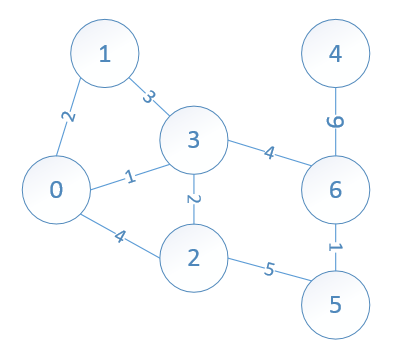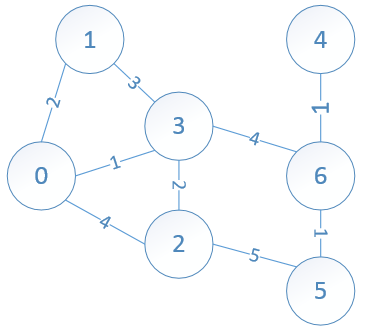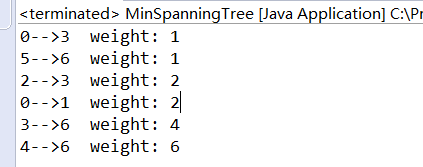0
0
01. 云栖社区>
2. 博客>
3. 正文

并查集与贪心算法的应用之求解无向图的最小生成树

1     private class Vertex {
2         private String vertexLabel;
3         private List<Edge> adjEdges;// 邻接表
4
5         public Vertex(String vertexLabel) {
6             this.vertexLabel = vertexLabel;
8         }
9     }

1 private class Edge implements Comparable<Edge> {
2         private Vertex startVertex;
3         private Vertex endVertex;
4         private int weight;// 边的权值
5
6         public Edge(Vertex start, Vertex end, int weight) {
7             this.startVertex = start;
8             this.endVertex = end;
9             this.weight = weight;
10         }
11
12         @Override
13         public int compareTo(Edge e) {
14
15             if (weight > e.weight)
16                 return 1;
17             else if (weight < e.weight)
18                 return -1;
19             else
20                 return 0;
21         }
22     }

文件格式如下：private Map<String, Vertex> nonDirectedGraph;

Kruskal算法是一个贪心算法，它与Dijkstra算法非常的相似。Kruskal算法贪心的地方在于：它总是选取图中当前权值最小的边的加入到树中（该边加入到树中之后不能出现环）。因此，这里就有个问题，如何选取当前权值最小的边？

Kruskal算法用到了并查集。因为，算法初始时将图中的各个顶点视为独立的，不连通的，随着一步步将当前权值最小的边加入，就将各个顶点连接起来了（使用并查集的Union操作实现连接）

①构造一个无向图啊。求解该图的最小生成树。----需要测试代码是否正确，得有一个实际的图。

②根据无向图中的顶点来 初始化 并查集----初始化过程和 并查集的应用之求解无向图中的连接分量个数 里面讲到的图的初始化过程一样。

③创建一个优先级队列来存储图中的边，这样每次选取边时，直接出队列，这比查找图中所有的边然后选择最小权值的边效率要高一点啊。

④判断这条边关联的两个顶点是否已经连通，如果已经连通了，再将该边加入到生成树中会导致环。

⑤直到所有的顶点都已经并入到生成树时，算法结束。

Kruskal算法用到并查集，那肯定需要实现并查集的基本操作，关于并查集，参考：数据结构--并查集的原理及实现

1     /**
2      *
3      * @param graph
4      *            求解graph的一棵最小生成树
5      * @return 组成最小生成树上的所有的边
6      */
7     public List<Edge> kruskal(Map<String, Vertex> graph) {
8         List<Edge> miniEdges = new ArrayList<Edge>(graph.size() - 1);
9
10         while (miniEdges.size() != graph.size() - 1) {
11             Edge e = pq.remove();
12             int start = Integer.valueOf(e.startVertex.vertexLabel);
13             int end = Integer.valueOf(e.endVertex.vertexLabel);
14             if (find(start) != find(end)) {
15                 union(start, end);
17             }
18         }
19         return miniEdges;
20     }1 import java.util.ArrayList;
4 import java.util.List;
5 import java.util.Map;
6 import java.util.PriorityQueue;
7
8 import c9.topo.FileUtil;
9
10 public class MinSpanningTree {
11     private class Vertex {
12         private String vertexLabel;
13         private List<Edge> adjEdges;// 邻接表
14
15         public Vertex(String vertexLabel) {
16             this.vertexLabel = vertexLabel;
18         }
19     }
20
21     private class Edge implements Comparable<Edge> {
22         private Vertex startVertex;
23         private Vertex endVertex;
24         private int weight;// 边的权值
25
26         public Edge(Vertex start, Vertex end, int weight) {
27             this.startVertex = start;
28             this.endVertex = end;
29             this.weight = weight;
30         }
31
32         @Override
33         public int compareTo(Edge e) {
34
35             if (weight > e.weight)
36                 return 1;
37             else if (weight < e.weight)
38                 return -1;
39             else
40                 return 0;
41         }
42     }
43
44     private Map<String, Vertex> nonDirectedGraph;
45     PriorityQueue<Edge> pq = new PriorityQueue<MinSpanningTree.Edge>();// 优先级队列存储边
46
47     private void buildGraph(String graphContent) {
48         String[] lines = graphContent.split("\n");
49
50         String startNodeLabel, endNodeLabel;
51         Vertex startNode, endNode;
52         for (int i = 0; i < lines.length; i++) {
53             String[] nodesInfo = lines[i].split(",");
54             startNodeLabel = nodesInfo;
55             endNodeLabel = nodesInfo;
56
57             endNode = nonDirectedGraph.get(endNodeLabel);
58             if (endNode == null) {
59                 endNode = new Vertex(endNodeLabel);
60                 nonDirectedGraph.put(endNodeLabel, endNode);
61             }
62
63             startNode = nonDirectedGraph.get(startNodeLabel);
64             if (startNode == null) {
65                 startNode = new Vertex(startNodeLabel);
66                 nonDirectedGraph.put(startNodeLabel, startNode);
67             }
68             Edge e = new Edge(startNode, endNode, Integer.valueOf(nodesInfo));
69             // 对于无向图而言,起点和终点都要添加边
72
74         }
75     }
76
77     private int[] s;// 存储并查集的一维数组
78
79     public MinSpanningTree(String graphContent) {
80         nonDirectedGraph = new LinkedHashMap<String, MinSpanningTree.Vertex>();
81         buildGraph(graphContent);
82
83         make_set(nonDirectedGraph);// 初始化并查集
84     }
85
86     private void make_set(Map<String, Vertex> graph) {
87         int size = graph.size();
88         s = new int[size];
89         for (Vertex v : graph.values()) {
90             s[Integer.valueOf(v.vertexLabel)] = -1;// 顶点的标识是从0开始连续的数字
91         }
92     }
93
94     private int find(int root) {
95         if (s[root] < 0)
96             return root;
97         else
98             return s[root] = find(s[root]);
99     }
100
101     private void union(int root1, int root2) {
102         if (find(root1) == find(root2))
103             return;
104         // union中的参数是合并任意两个顶点,但是对于并查集,合并的对象是该顶点所在集合的代表顶点(根顶点)
105         root1 = find(root1);// 查找顶点root1所在的子树的根
106         root2 = find(root2);// 查找顶点root2所在的子树的根
107
108         if (s[root2] < s[root1])// root2 is deeper
109             s[root1] = root2;
110         else {
111             if (s[root1] == s[root2])// 一样高
112                 s[root1]--;// 合并得到的新的子树高度增1 (以root1作为新的子树的根)
113             s[root2] = root1;// root1 is deeper
114         }
115     }
116
117     /**
118      *
119      * @param graph
120      *            求解graph的一棵最小生成树
121      * @return 组成最小生成树上的所有的边
122      */
123     public List<Edge> kruskal(Map<String, Vertex> graph) {
124         List<Edge> miniEdges = new ArrayList<Edge>(graph.size() - 1);
125
126         while (miniEdges.size() != graph.size() - 1) {
127             Edge e = pq.remove();
128             //生成并查集操作的对应的标点位置
129             int start = Integer.valueOf(e.startVertex.vertexLabel);
130             int end = Integer.valueOf(e.endVertex.vertexLabel);
131             if (find(start) != find(end)) {
132                 union(start, end);
134             }
135         }
136         return miniEdges;
137     }
138
139     // for test purpose
140     public static void main(String[] args) {
141         String graphFilePath;
142         if (args.length == 0)
143             graphFilePath = "F:\\graph.txt";
144         else
145             graphFilePath = args;
146
147         String graphContent = FileUtil.read(graphFilePath, null);// 从文件中读取图的数据
148         MinSpanningTree mst = new MinSpanningTree(graphContent);
149
150         // 获得图中组成最小生成树的所有边
151         List<Edge> edges = mst.kruskal(mst.nonDirectedGraph);
152         for (Edge edge : edges) {
153             System.out.print(edge.startVertex.vertexLabel + "-->"
154                     + edge.endVertex.vertexLabel);
155             System.out.println("  weight: " + edge.weight);
156         }
157     }
158 }

FileUtil类可参考中的完整代码实现。

0-->3 表示顶点0到顶点3的边，边的权值为1

5-->6 表示顶点5到顶点6的边，边的权值为1

.......+ 关注

corcosa 12349人浏览